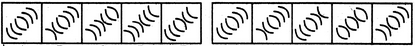# Non Verbal Reasoning - Series - Discussion

Discussion Forum : Series - Section 1 (Q.No. 14)
Directions to Solve

Each of the following questions consists of five figures marked A, B, C, D and E called the Problem Figures followed by five other figures marked 1, 2, 3, 4 and 5 called the Answer Figures. Select a figure from amongst the Answer Figures which will continue the same series as established by the five Problem Figures.

14.

Select a figure from amongst the Answer Figures which will continue the same series as established by the five Problem Figures.(A)     (B)     (C)     (D)     (E)       (1)     (2)     (3)     (4)     (5)
1
2
3
4
5
Explanation:
One arc and four arcs get inverted alternately.
Discussion:
10 comments Page 1 of 1.

Kunal said:   3 years ago
Thanks @Swathi.

Priyanka said:   3 years ago
Thanks @Shalini.

Shankar said:   4 years ago
Thanks @Manan.

Ilcan said:   5 years ago
Number 1 is inverted.
2,3,4,5 are inverted.
6 is inverted.
1234 are inverted.
So, 5 should get inverted.
(2)

Vandana Harish said:   5 years ago
If 1st then next four, then 1st from last then first 4, then next should be 1st from the beginning? then how come figure 3? Please explain the answer in detail.

Fig B -1st arc changed.
FigC-2345 changed.
Since 6th is left so 6th will change.
FigD -6th changed. FigE-1234 changed.
Hence, 5th is left so 5th will change.
(2)

Manan said:   10 years ago
Let's say that the top arc is the 1st arc and the bottom most arc is the 6th one. According to me A & B, C & D and E & F (where F is the answer) are pairs with slight change.

If we look at A & B figures, there's only one change in B i.e. only 1 arc gets inverted (6th arc).

Now move onto C & D figures. Here also only one arc gets inverted (1st arc).

Now the hint which comes up is that the Answer should be that one where only one arc gets inverted.

And only C gives that (only 2nd arc is inverted).